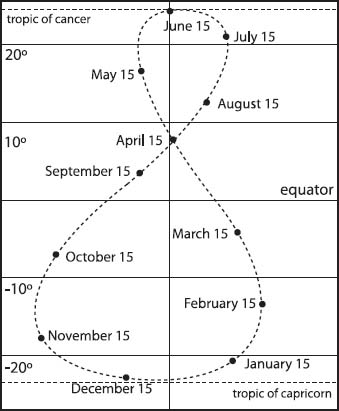NextPrevious

# How do astronomers determine the distances to other planets?

Astronomers need mathematics, of course, to determine the distances to the planets and satellites of our solar system. One of the first astronomers to work this out was Nicolaus Copernicus (see above) using simple observations of planetary positions.If you carefully chart the position of the Sun at the same time of day over the course of a year, at the mid-latitudes you will see that it appears to follow a figure eight pattern in the sky.

One of the earliest methods to determine such distances was to use the orbital period of a planet. This varies as the square root of the cube of the distance from the Sun: T = k × r(3/2), in which T is the time for one revolution, r is the distance between the centers of the Sun and the planet, and k is a constant.

Close

This is a web preview of the "The Handy Math Answer Book" app. Many features only work on your mobile device. If you like what you see, we hope you will consider buying. Get the App